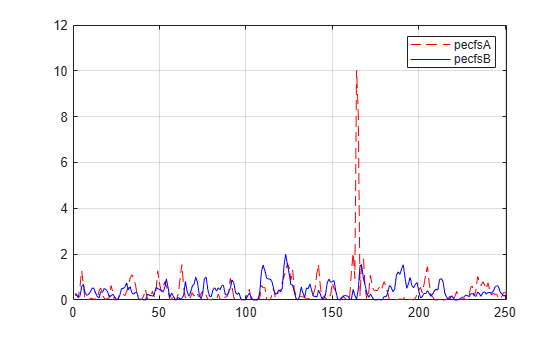# wdecenergy

Multisignal 1-D decomposition energy distribution

## Syntax

``[e,pec,pecfs] = wdecenergy(dec)``
``[e,pec,pecfs,idxsort,longs] = wdecenergy(dec,"sort")``
``[e,pec,pecfs] = wdecenergy(dec,optsort,idxsig)``
``[e,pec,pecfs,idxsort,longs] = wdecenergy(dec,optsort,idxsig)``

## Description

example

````[e,pec,pecfs] = wdecenergy(dec)` returns the energy `e` (L2-norm) of each decomposed signal `dec`, the percentage of energy `pec` for each wavelet component (approximation and details) of each signal, and the percentage of energy `pecfs` for each coefficient.```
````[e,pec,pecfs,idxsort,longs] = wdecenergy(dec,"sort")` returns `pecfs` sorted (by row) in ascending order and an index vector `idxsort`. `longs` contains the lengths of each family of coefficients. Replacing `"sort"` by `"ascend"` returns the same result.Replacing `"sort"` by `"descend"` returns `pecfs` sorted in descending order. ```
````[e,pec,pecfs] = wdecenergy(dec,optsort,idxsig)` returns the values for the signals whose indices are given by `idxsig`.```
````[e,pec,pecfs,idxsort,longs] = wdecenergy(dec,optsort,idxsig)` also returns the indices `idxsort` and lengths of each family of coefficients `longs`.```

## Examples

collapse all

Load the 23 channel EEG data `Espiga3` . The channels are arranged column-wise. The data is sampled at 200 Hz.

`load Espiga3`

Perform a decomposition at level 2 using the `db2` wavelet.

`dec = mdwtdec("c",Espiga3,2,"db2")`
```dec = struct with fields: dirDec: 'c' level: 2 wname: 'db2' dwtFilters: [1x1 struct] dwtEXTM: 'sym' dwtShift: 0 dataSize: [995 23] ca: [251x23 double] cd: {[499x23 double] [251x23 double]} ```

Compute the energy distribution.

`[e,pec,pecfs] = wdecenergy(dec);`

Display the total energy and the distribution of energy for each wavelet component (A2, D2, D1) in the second channel.

```idx = 2; e(idx)```
```ans = 8.0761e+05 ```
`perA2D2D1 = pec(idx,:)`
```perA2D2D1 = 1×3 99.0583 0.8535 0.0882 ```

Compare the coefficient energy distribution for signal 1 and signal 10. Because most of the energy is in the approximation coefficients, zoom in the x-axis by the number of approximation coefficients.

```sigA = 1; sigB = 10; pecfsA = pecfs(sigA,:); pecfsB = pecfs(sigB,:); plot(pecfsA,"r--") hold on plot(pecfsB,"b") hold off grid on legend("pecfsA","pecfsB") xlim([0 size(dec.ca,1)])```## Input Arguments

collapse all

Wavelet decomposition, specified as a structure. `dec` is the output the `mdwtdec`. `dec` has the following fields:

• `dirDec` — Direction indicator: `'r'` (row) or `'c'` (column)

• `level` — Level of wavelet decomposition

• `wname` — Wavelet name

• `dwtFilters` — Structure with four fields containing the filter coefficients: `LoD`, `HiD`, `LoR`, and `HiR`

• `dwtEXTM` — DWT extension mode

• `dwtShift` — DWT shift parameter (0 or 1)

• `dataSize` — Size of the multisignal whose decomposition is `dec`.

• `ca` — Approximation coefficients at level `level`

• `cd` — Cell array of detail coefficients, from level 1 to level `level`

The coefficients `ca` and `cd{k}`, for k from 1 to `level`, are matrices and are stored in rows if `dirdec = 'r'` or in columns if `dirdec = 'c'`. For more information, see `mdwtdec`.

Sorting method to apply to `pecfs`, specified as one of the following values:

• `"none"` — No sorting

• `"sort"` — Ascending

• `"ascend"` — Ascending

• `"descend"` — Descending

Signal indices, specified as a real-valued vector. The `wdecenergy` function returns the values for the signals specified by `idxsig`.

## Output Arguments

collapse all

Energy (L2-norm) of each decomposed signal in `dec`, returned as a real-valued vector. `e(i)` is the energy of the ith signal.

Percentage of energy for each wavelet component (approximation and details) of each signal.

• `pec(i,1)` is the percentage of energy for the approximation of level `maxlev = dec.level` of the ith signal.

• `pec(i,j)`, for `j=2,...,maxlev+1`, is the percentage of energy for the detail of level `(maxlev+1-j)` of the ith signal.

Percentage of energy for each coefficient, returned as a real-valued matrix. `pecfs(i,j)` is the percentage of energy for jth coefficient of the ith signal.

Index vector for the sorted matrix `pecfs`.

Lengths of each family of coefficients, returned as a vector.

 Mesa, Hector. “Adapted Wavelets for Pattern Detection.” In Progress in Pattern Recognition, Image Analysis and Applications, edited by Alberto Sanfeliu and Manuel Lazo Cortés, 3773:933–44. Berlin, Heidelberg: Springer Berlin Heidelberg, 2005. https://doi.org/10.1007/11578079_96.